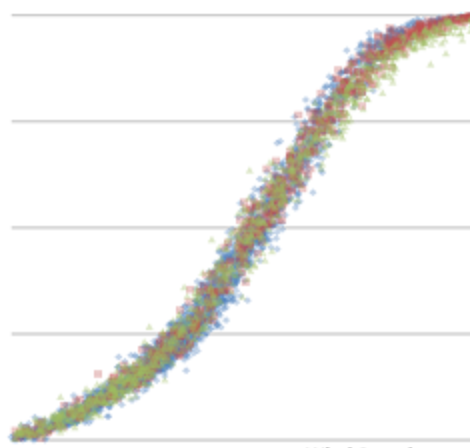#The Power Curve

If the bell curve is the underlying paradigm of the industrial economy, the power curve is the model that defines our new tech-enabled creative economy.Power Curve

It all started with Moore's Law that predicted the exponential growth of the computational power of computers.

Then there is Koomey's Law that predicted the exponential shrinking of the battery size, and thus the decreasing size of electronic devices.

And then there is Reed's Law that predicted exponential increase in the size of social networks that connect all of us together.

The power curve defines our new economy. An economy that is driven by innovation that is increasing at an exponentially faster rate.

My challenge, then, became modeling the power curve in the classroom.

DOT FROM preview-next-diagram